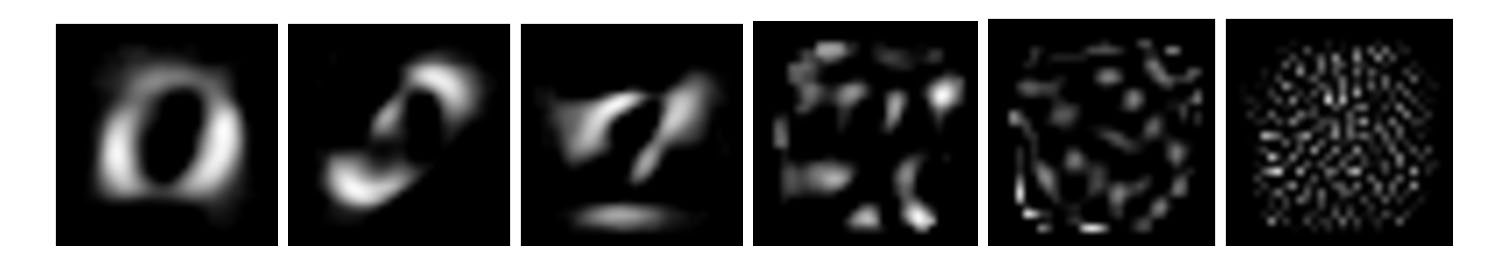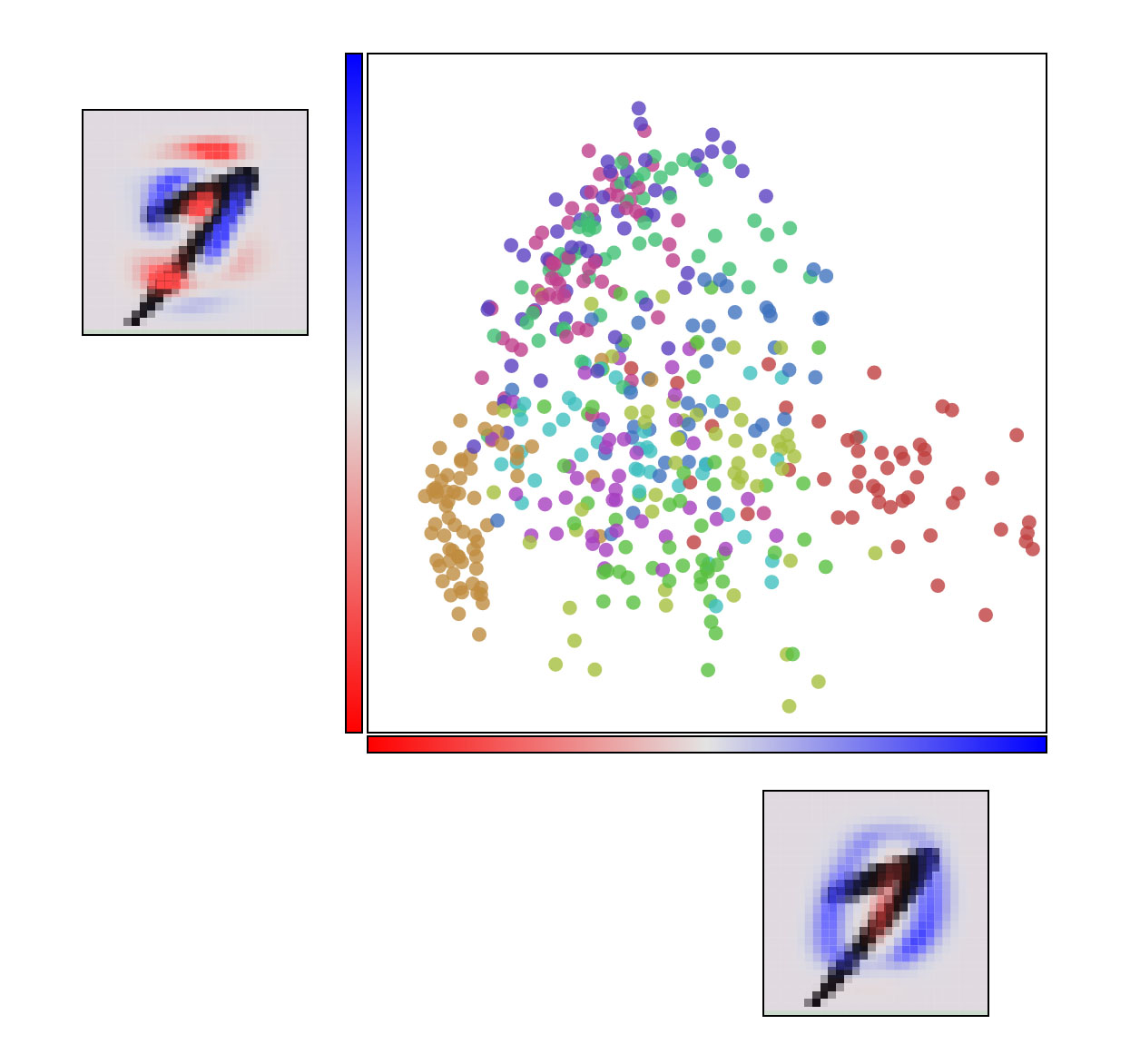Written by Justin Girard, former Westie

## Classification of Handwritten Numbers

When trying to get a grasp on how machine learning (ML) works, it is often useful to experiment with existing ML problems. One popular starting point is the MNIST database (Modified National Institute of Standards and Technology), a collection of image scans of handwritten numbers by clerics and high school students. If you look at the MNIST TensorFlow demo, we see an introductory approach to detecting handwritten digits with 91% accuracy. The general problem is this, given a digit, we would like to predict if it is a real number from 0-9.A simple sequence may be interpreted as “5041” by a well trained classifier. In the TensorFlow example, however, the data is labelled; this means each training-digit has a corresponding “value” of 0-9 assigned, so the classifier, in this case a softmax regressor, is told what output is expected. This is called supervised.

## Principal Component Analysis (PCA) Of The MNIST Data Set

Now, a contrary domain is unsupervised learning. This problem is much more difficult, and in the MNIST space, can be described as detecting how many kinds of symbols there are. In a human sense, this would be akin to guessing all the kinds of symbols in an alien language. Thus, our unsupervised goal is to have our system discover the different classes of digits (0-9) by itself!

We decided that a two-step approach would be appropriate to accomplish this. Discussed here is the first step: running the MNIST data through a PCA algorithm.

## PCA Introduction

PCA (Principal Component Analysis) is a process of reducing the dimensions of a set of data while trying to maintain statistically significant information on that set. For example, if we had a 2-dimensional set of data (x,y), we would try to find some new axis on which this data’s original meaning is somewhat preserved. Broadly, this can be coined dimensionality reduction, but in another sense projecting data onto a new axis can lead to a more separable set of data. (We will ignore the extremely useful kernel trick here.)

## PCA Implementation

There are many tutorials about how to apply PCA to MNIST, but it is worthwhile discussing what exactly the discovered eigenvectors represent. In essence, from left to right, the presence of the vectors signify the most variance in the dataset. So a shape that looks something like an 0 distinguishes many of the characters (0-9), then second, a symbol that appears to only model the top and bottom of a seven or two. In a real sense, a linear combination of the first four eigenvectors can create any human readable number. The last selling point of PCA, is that the late vectors, with smaller eigenvalues, may only model noise or random perturbations.## Clustering Implementation

After dimensionality reduction, we may theorize that the data, at least along the first principal components, is separable. Essentially, below, each color is a single number, and thus we can see that this manner the number one (yellow) is likely to fall in a different cluster than that of a zero (red).Overall, there are many approaches to applying unsupervised and supervised learning to the MNIST dataset. In this sense, it is possible to discover new and interesting features from existing datasets. It’s recommended to jump into some of the introductory blogs available.